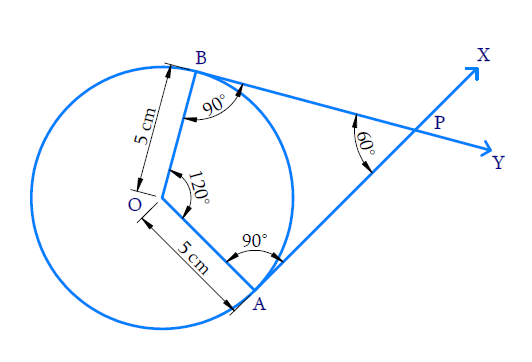# Ex.11.2 Q4 Constructions Solution - NCERT Maths Class 10

Go back to  'Ex.11.2'

## Question

Draw a pair of tangents to a circle of radius $$5 \,\rm{cm}$$ which are inclined to each other at an angle of $$60^\circ$$.

## Text Solution

#### Steps:Steps of construction:

(i)    With $$O$$ as centre and $$5 \,\rm{cm}$$ as radius draw a circle.

(ii)    Take a point $$A$$ on the circumference of the circle and join $$OA$$.

(iii)   Draw $$AX$$ perpendicular to $$OA$$.

(iv)   Construct \begin{align}\angle \rm{AOB}=120^{\circ} \end{align} where $$B$$ lies on the circumference.

(v)    Draw $$BY$$ perpendicular to $$OB$$.

(vi)   Both $$AX$$ and $$BY$$ intersect at $$P$$.

(vii)  $$PA$$ and $$PB$$ are the required tangents inclined at \begin{align}60^{\circ}\end{align} .

Proof:

$$\angle {{OAP}} = \angle {{OBP}} = 90^\circ$$ (By construction)

$$\angle {{AOB}} = 120^\circ$$ (By construction)

In quadrilateral $$OAPB$$,

\begin{align} \angle {{APB}}& = 360^\circ - [\angle {{OAP}} + \angle {{OBP}} + \angle {{AOB}}]\\ &= 360^\circ - [90^\circ + 90^\circ + 120^\circ ]\\ &= 360^\circ - 300^\circ \\ &= 60^\circ \end{align}

Hence $$PA$$ and $$PB$$ are the required tangents inclined at \begin{align}60^{\circ}\end{align}.

Learn from the best math teachers and top your exams

• Live one on one classroom and doubt clearing
• Practice worksheets in and after class for conceptual clarity
• Personalized curriculum to keep up with school